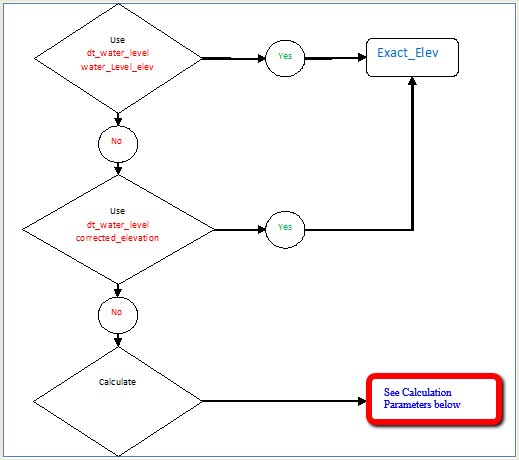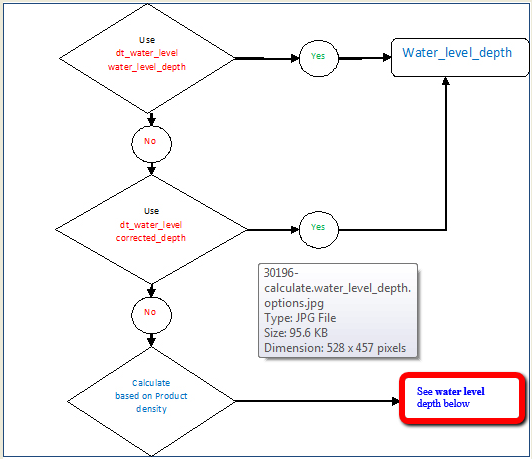﻿ Water Level Report Basics

Water Level Report Basics

EQuIS Library > Reports > EarthSoft Standard Reports > Water Level Reports >

Water Level Report Basics

The Water Levels (db function) and Water Levels II (Class) Reports are included in the standard suite of reports provided with EQuIS Professional and EQuIS Enterprise. The Water Level Reports return the field measured water level elevations as stored directly in EQuIS or as calculated or estimated water level elevation based on user inputs, if LNAPL thickness and density are stored in the database. This article will instruct you on how to use the report, what options are available within the report, and what data are required to complete each of the options.

To open the Water Level Reports in EQuIS Professional:

1.Select Reports from the Open section of the Home ribbon to open the Reports window.

2.Select Water Levels or Water Levels II and hit Enter or double-click to open one or both reports.

Water Level Reports - Overview

The Water Level Reports return water level data based on selected parameters. The report is capable of calculating estimated water levels for wells that contain light non-aqueous phase liquid (LNAPL), because free product present within a monitoring well depresses the water level such that the measured water level is not representative of the true water level within the ground. The report includes three fields to which all other metadata can be related:

1.SYS_LOC_CODE: name of sampling location

2.MEASUREMENT_DATE: date and time that water level measurement was obtained

3.Water Level Elevation: the Water Level Report returns the following two main fields and others:

1.EXACT_ELEV: water level elevation based on Reported Value parameter inputs, expressed as a numeric value

2.WATER_LEVEL: water level elevation based on Reported Value parameter inputs, expressed as a text field, maintaining the number of significant figures

Data Requirements and Options

The DEPTH_UNIT field must be populated in DT_WELL, DT_WATER_LEVEL and/or DT_FACILITY in order for the report to return the water level elevations associated with the selected locations.

Data for the Water Level Report is extracted primarily from DT_WATER_LEVEL. This data table may be found by selecting Data Tables and then selecting DT_WATER_LEVEL. To accommodate various methodologies in collecting and storing water level data, there are four different fields to store the water level information in DT_WATER_LEVEL:

1.WATER_LEVEL_DEPTH: the measured depth to water from a reference point on a well. The value is entered by the user and stored in the database.

2.WATER_LEVEL_ELEVATION: the measured elevation of water above a reference point; usually meters or feet above sea level. This value may be stored in the database or calculated by EQuIS when the Water Level Report is generated.

3.CORRECTED_DEPTH: the measured depth to water corrected for LNAPL presence, if necessary, by the user prior to import.

4.CORRECTED_ELEVATION: the measured water level elevation corrected for LNAPL presence, if necessary, by the user prior to import.

Within DT_WATER_LEVEL there is also an additional field that allows you to specify whether the recorded water level was above or below the reference point:

DIP_OR_ELEVATION: Dip is populated when the measured water level is below the reference elevation. Elevation is used where the measured water level is above the reference elevation, e.g. height of water above a transducer, above a sea bed or above an artesian well. The concept of Dip and Elevation is illustrated in Figure 2.Figure 2: Illustration of Dip and Elevation Concepts as used in EQuIS

Reported Value Parameter

The Reported Value Parameter includes three options for user selection:1.water_level_elev: This is the default selection, which populates WATER_LEVEL and EXACT_ELEV values directly from DT_WATER_LEVEL.WATER_LEVEL_ELEV (Figure 3).

2.corrected_elevation: This option populates the WATER_LEVEL output column directly from DT_WATER_LEVEL.CORRECTED_ELEVATION.

For the above two Reported Value input selections, the Calculation\Elevation and/or Calculation\Depth parameters are not used.

calculate: When this option is selected, the water_level and exact_elev may be calculated based on the Calculation\Elevation and Calculation\Depth parameter selections as explained below.Figure 3

Calculation Parameters

The following calculation for the water level elevation is performed based on dip_or_elevation values, the selected Elevation parameter, which determines the reference_elev, and the selected Calculation\Depth parameter which determines the water_depth:

 EXACT_ELEV = REFERENCE_ELEV - WATER_DEPTH when DIP_OR_ELEVATION = dip                           or                        = REFERENCE_ELEV + WATER_DEPTH when DIP_OR_ELEVATION = elevation

The values for reference elev and water depth can be collected, stored, and calculated using various methodologies as stated in the following.

Elevation

Report Output Column: REFERENCE_ELEV: The user selection can be one of four options, explained below.1.historical_reference_elev: This option populates REFERENCE_ELEV directly from DT_WATER_LEVEL.HISTORICAL_REFERENCE_ELEV.

2.measure_datum: If this option is selected, the REFERENCE_ELEV output is populated from DT_MEASURE_DATUM.DATUM_VALUE and DT_MEASURE_DATUM.STEP_OR_LINEAR to take into account the potential for a change in the elevation of the top of the well. The elevation of the top of the well may change due to many circumstances, including any of the following examples:

i.a gradual change in ground level (e.g. degassing and subsidence at a landfill) or

ii.a sudden change (e.g. a truck hitting the well)

The elevation value is entered in DT_MEASURE_DATUM.DATUM_VALUE and DT_MEASURE_DATUM.STEP_OR_LINEAR should be completed with a description of whether the elevation change was a step or linear change, examples of which are as follows:

3.  Linear - in the case of the landfill, the reference elevation was measured on 1st Jan and equaled 200 ft. Then, on returning on 1st June, it was re-measured to 199 ft, representing a gradual change in the elevation. If you ran a Water Level Report for 15 March, it would return a MEASURE_DATUM value of 199.5 ft. After 1st June, it would return 199 ft.

For Linear, note the output considers DT_MEASURE_DATUM with start_date prior to and closest to (or before, call it as A) and after and closest to measurement_date (call it as B) in the calculations: reference_elev = A + (B-A) / (B_start_date - A_start_date) * (measurement_date - A_start_date).

4.  Step - a sudden elevation change, such as a truck damaging the well stickup, the reference elevation on 1st Jan was 200 ft, but on 1st June, the truck hits and knocks 1 ft off the well. Running a report for data on 15 March would return a MEASURE_DATUM of 200 ft, however, running a report for 2nd June would return an elevation of 199 ft.

For Step, if a location has more than one start_date record in DT_MEASURE_DATUM, the report returns one record per location where the DT_MEASURE_DATUM.START_DATE is the nearest date preceding the DT_WATER_LEVEL.MEASUREMENT_DATE.

surf_elev: This option populates REFERENCE_ELEV from vw_location.surf_elev, which maps to DT_COORDINATE.ELEV.

top_casing_elev: This option populates REFERENCE_ELEV directly from the database: DT_WELL.TOP_CASING_ELEV.

Depth (Report Output Column: WATER_DEPTH): The user selection can be one of three options, explained below and shown in Figure 4.corrected_depth: WATER_DEPTH will be DT_WATER_LEVEL.CORRECTED_DEPTH directly retrieved from the database (Figure 4)

water_level_depth: WATER_DEPTH populates from DT_WATER_LEVEL. WATER_LEVEL_DEPTH (Figure 4)

calculate: WATER_DEPTH is calculated from Product Thickness as described below.Figure 4

water_depth = DT_WATER_LEVEL.WATER_LEVEL_DEPTH - lnapl_density * (DT_WATER_LEVEL.WATER_LEVEL_DEPTH - lnapl_depth) or

= DT_WATER_LEVEL.WATER_LEVEL_DEPTH if lnap_depth is not available.

 Note: As stated above, water_depth is determined by the Calculation\Depth parameter which is not available when the Water Level\Reported Value parameter is not selected as calculate in the Water Levels II. If needed, users may select Water Level\Reported Value as calculate first, select Calculation\Depth, and then change Water Level\Reported Value back to intended values.

The above describes how reference_elev, water_level, exact_level and water_depth of the report output are generated. The other non-direct or calculated outputs are introduced below.

Depth_unit: determined in the order of DT_FACILITY.ELEV_UNIT, DT_WELL.DEPTH_UNIT, and DT_WATER_LEVEL.DEPTH_UNIT

lnapl_thickness: determined by DT_WATER_LEVEL.WATER_LEVEL_DEPTH - lnapl_depth

dnapl_thickness: determined by measured_depth_of_well - dnapl_depth

lnapl_density: determined in the order of the Water Level\User-estimated product density: parameter (hidden) or RT_ANALYLTE_DETAIL.SPECIFIC_GRAVITY where cas_rn = DT_WATER_LEVEL.LNAPL_CAS_RN or the Water Level\Default Specific Gravity: parameter (hidden, defaults to 0.8). Note that the hidden parameters can be made visible and editable to the user after the report is published to ST_REPORT.

Unit Conversion: The Water Level Report will not return reference_elev, water_level and exact_level data if the associated unit is null. The user must populate either DT_WATER_LEVEL.DEPTH_UNIT, DT_FACILITY.ELEV_UNIT or DT_WELL.DEPTH_UNIT for water level data to display in the Water Level Report. Also, the report will not return values if the units used do not have a conversion factor listed in RT_UNIT_CONVERSION_FACTOR for both ways (e.g., ft to ft bgs and ft bgs to ft).

Unit conversion for reference_elev, water_level, exact_level:

Here is how the original unit of each Calculation\Elevation parameter selection is retrieved:

top_casing_elev: in the order of DT_WELL.DEPTH_UNIT,DT_WATER_LEVEL.DEPTH_UNIT,DT_FACILITY.ELEV_UNIT

measure_datum: DT_MEASURE_DATUM.DATUM_UNIT,DT_WATER_LEVEL.DEPTH_UNIT,DT_WELL.DEPTH_UNIT,DT_FACILITY.ELEV_UNIT

surf_elev: vw_location.elev_unit,DT_WATER_LEVEL.DEPTH_UNIT,DT_WELL.DEPTH_UNIT,DT_FACILITY.ELEV_UNIT

historical_reference_elev: DT_WATER_LEVEL.DEPTH_UNIT,DT_WELL.DEPTH_UNIT,DT_FACILITY.ELEV_UNIT

the original unit is converted to: DT_FACILITY.ELEV_UNIT,DT_WELL.DEPTH_UNIT or DT_WATER_LEVEL.DEPTH_UNIT in order.

If DT_MEASURE_DATUM is selected for the Calculation>Elevation parameter, any reported REFERENCE_ELEVs for MEASUREMENT_DATEs that are prior to the START_DATE set in DT_MEASURE_DATUM will use DT_WATER_LEVEL.HISTORICAL_REFERENCE_ELEV for the report REFERENCE_ELEV field.

Unit conversion for measured_depth_of_well, water_depth, lnapl_depth, lnapl_thickness, dnapl_depth:

from the original unit of DT_WATER_LEVEL.DEPTH_UNIT, DT_WELL.DEPTH_UNIT or DT_FACILITY.ELEV_UNIT to the output unit of DT_FACILITY.ELEV_UNIT, DT_WELL.DEPTH_UNIT or

DT_WATER_LEVEL.DEPTH_UNIT in order.